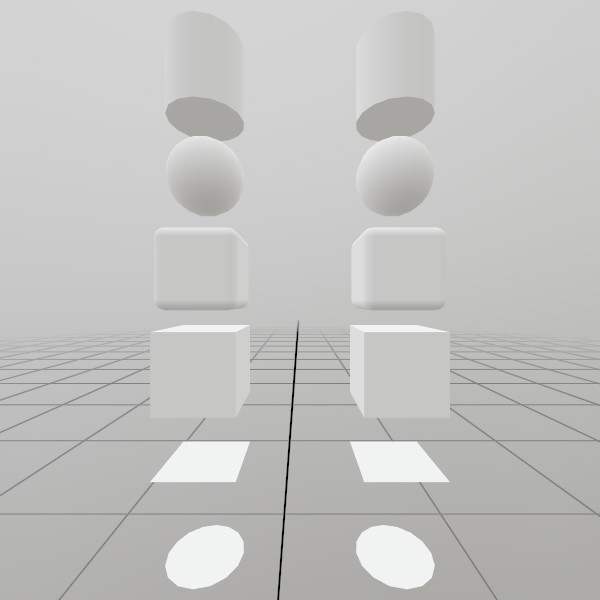# Mesh.GenerateCube

static Mesh GenerateCube(Vec3 dimensions, int subdivisions)

Vec3 dimensions How large is this cube on each axis, in meters?
int subdivisions Use this to add extra slices of vertices across the cube’s faces. This can be useful for some types of vertex-based effects!
RETURNS: Mesh A flat-shaded cube mesh, pre-sized to the given dimensions.

Generates a flat-shaded cube mesh, pre-sized to the given dimensions. UV coordinates are projected flat on each face, 0,0 -> 1,1.

## ExamplesHere’s a quick example of generating a mesh! You can store it in just a Mesh, or you can attach it to a Model for easier rendering later on.

``````Mesh  cubeMesh  = Mesh.GenerateCube(Vec3.One * 0.4f);
Model cubeModel = Model.FromMesh(cubeMesh, Default.Material);
``````

Drawing both a Mesh and a Model generated this way is reasonably simple, here’s a short example! For the Mesh, you’ll need to create your own material, we just loaded up the default Material here.

``````Matrix cubeTransform = Matrix.T(-1.0f, 0, 1);